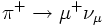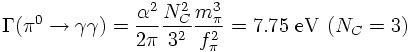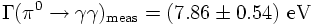## QCD

### Why Colour?

There are several strong evidences why quarks should carry - besides the electric charge - an additional internal degree of freedom: the colour charge.
1. Quark Wave Function of Baryons
2. Electron Positron Annihilation into Hadrons
3. tau decays
4. pi0 decay
The dominant decay of a neutral pion is the electromagnetic decay in two photons: BF = 98.8 percent.

Sideremark: Since the decay into three photons is not observed and electromagnetic interactions conserve charge conjugation C one concludes that the neutral pion is an eigenstate of C with eigenvalue +1.

According to the quark model the flavor state of the pion in terms of quark states is given by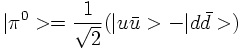The leading order Feyman diagrams for the pion decay into two photons due to quark antiquark annihilation is given by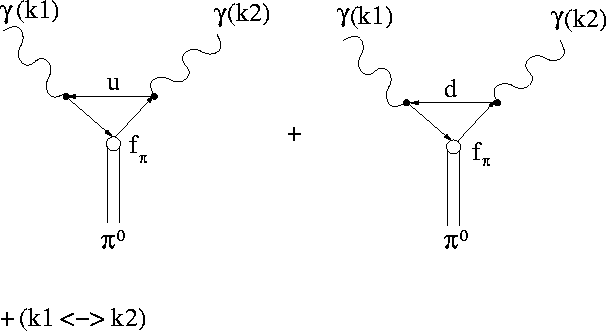The blob means that the quark-antiquark pair is in a bound state.

The matrix element is proportional to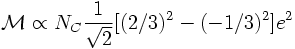More precisely, the matrix element is given by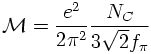where fπ is the pion decay constant which is a measure of the probability that the quark and antiquark are found at the origin. The pion decay constant can be measured in the leptonic decay of charged pions